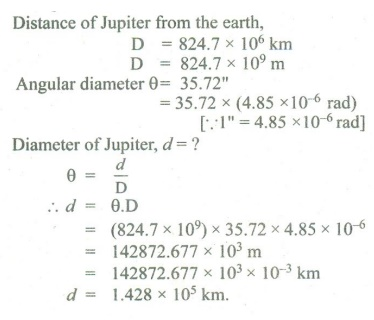Home | | Physics 11th std | Book Back Numerical Problems

# Book Back Numerical Problems

Physics : Nature of Physical World and Measurement : Book Back Exercise, Example Numerical Question with Answers, Solution : Book Back Numerical Problems

## 1. In a submarine equipped with sonar, the time delay between the generation of a pulse and its echo after reflection from an enemy submarine is observed to be 80 sec. If the speed of sound in water is 1460 ms-1. What is the distance of enemy submarine?Answers:The speed of sound in water v = 1460 ms-1Time taken by the pulse for to and fro :t = T/2 = 80s/2 = 40sv = d/td = v × T/2 = 1460 × 40= 58400 m or 58.40 km.Ans: (58.40 km)2. The radius of the circle is 3.12 m. Calculate the area of the circle with regard to significant figures.Answers:Radius of the circle r = 3.12 mArea of the circle A = ?A = πr2 = 3.14 × 3.12 × 3.12 = 30.566016According to the rule of significant fig,A = 30.6 m2 [Given data has three sig. fig.]Ans: (30.6 m 2)3. Assuming that the frequency γ of a vibrating string may depend upon i) applied force (F) ii) length (l) iii) mass per unit length (m), prove that γausing dimensional analysis.Answers:γ ∝ lm Fb mcγ = K lm Fb mcK - dimensionless constant of proportionalitya,b,c - powers of l, F, mDimensional Formula of F = [MLT-2]Dimensional Formula of linear densityApplying the principle of homogeneity of dimensionb + c = 0     ....(1)a + b -c = 0 ….. (2)4. Jupiter is at a distance of 824.7 million km from the Earth. Its angular diameter is measured to be 35.72˝. Calculate the diameter of Jupiter.Ans: (1.428 × 105 km)5. The measurement value of length of a simple pendulum is 20 cm known with 2 mm accuracy. The time for 50 oscillations was measured to be 40 s within 1 s resolution. Calculate the percentage accuracy in the determination of acceleration due to gravity ‘g’ from the above measurement.Answers:The errors in both Z & T are least count errors.Ans: (6%)

Tags : Nature of Physical World and Measurement | Physics , 11th Physics : UNIT 1 : Nature of Physical World and Measurement
Study Material, Lecturing Notes, Assignment, Reference, Wiki description explanation, brief detail
11th Physics : UNIT 1 : Nature of Physical World and Measurement : Book Back Numerical Problems | Nature of Physical World and Measurement | Physics